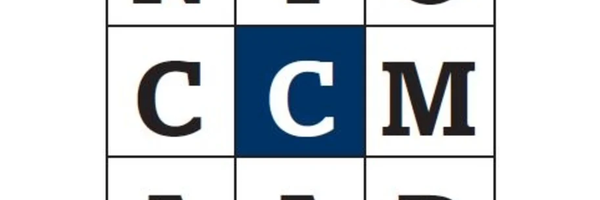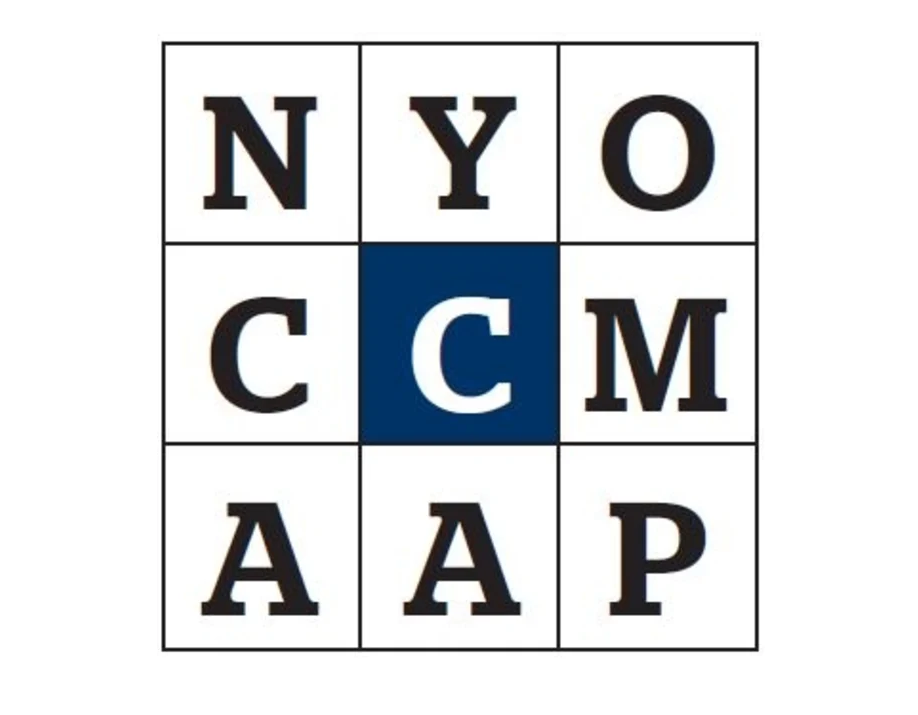# The Target puzzle: searching wordlists with tries

I was thinking about the Target puzzle from a certain Australian newspaper the other day: you're given 9 letters, and you have to create as many words as possible from them (length 4+). There's always at least one 9 letter word you can form.This is going to be a short post, as I basically wanted to check whether you could use a trie to solve this.

Tries are a way of storing strings (or other sequences) so they can be searched quickly: basically, you build a tree where each letter in the string becomes a node, with the next letter in the string becoming a child node. The time to search for a string of length $$m$$ is $$O(m)$$.

A simple trie storing a small list of words looks like:

def build_trie(words):
trie = {}
for word in words:
current_dict = trie
for index, c in enumerate(word):
if index == (len(word) - 1):
current_dict[c] = True
else:
if c not in current_dict:
current_dict[c] = {}
current_dict = current_dict[c]
return trie

simple_trie = build_trie(['act', 'bat', 'bag', 'bin'])
print(simple_trie)

{'a': {'c': {'t': True}}, 'b': {'a': {'t': True, 'g': True}, 'i': {'n': True}}}


Note that here, we're just looking for 9 letter words, so I just assume each word is equal length and terminate the branches with a True.

To check if a word occurs in the trie we start at the root, then for each letter in the word, we check if that letter is a child of the current node. If it is, we step down to that node and move to the next letter:

def word_in_trie(word, trie):
current_dict = trie
for letter in word:
if not letter in current_dict:
return False
current_dict = current_dict[letter]
return True

word_in_trie('bat', simple_trie)

True


To solve the Target puzzle, we'll use the standard word list built into the OS, and grab all the 9 letter words. We'll create a trie, as well as a standard word list that we'll use when comparing the trie approach to alternatives.

def generate_words(filename, word_length=9):
with open(filename) as word_list:
for line in word_list:
word = line.strip().lower()
if len(word) == word_length:
if word.isalpha():
yield word

word_trie = build_trie(generate_words('/usr/share/dict/words'))
word_list = list(generate_words('/usr/share/dict/words'))

word_list[:3]

['abernathy', 'abyssinia', 'accenture']


To find a 9-letter word using the trie approach, we just generate permutations of the available letters, and check if any of them form a full word:

from itertools import permutations

def solve_target(letters, trie):
letters = list(c.lower() for c in letters)
for comb in permutations(letters, 9):
if word_in_trie(comb, trie):
return ''.join(comb)

solve_target('WDPARUPNE', word_trie)

'unwrapped'


This seems pretty fast, especially given that it's potentially having to run through a large number of permutations:

sum(1 for comb in permutations('WDPARUPNE'))

362880


If my (admittedly fuzzy) knowledge of big-O notation is right, the runtime for this trie-based search should be something like $$O(pm)$$ where $$p$$ is the number of permutations, and $$m$$ is the length of the words ($m = 9$ here).

Unfortunately when I tested it against a set based implementation, it seems like the set wins out:

def solve_with_set(letters, word_set):
letters = list(c.lower() for c in letters)
for comb in permutations(letters, 9):
if comb in word_set:
return ''.join(comb)

%%timeit -n 10 -r 1
solve_target('WDPARUPNE', word_trie)

62 ms ± 0 ns per loop (mean ± std. dev. of 1 run, 10 loops each)

%%timeit -n 10 -r 1
solve_with_set('WDPARUPNE', set(tuple(w) for w in word_list))

28.6 ms ± 0 ns per loop (mean ± std. dev. of 1 run, 10 loops each)


The set-based solution should also have a worst-case runtime of $O(pn)$, where $n$ is the total number of words in the list, which should mean our trie wins out as the word list gets longer and longer. However, set lookups are often $O(1)$, and the Python implementation of them is pretty efficient, so we might only see the advantages of tries for this kind of puzzle under specific circumstances. I might have to put this down as an idea that didn't quite pay off.